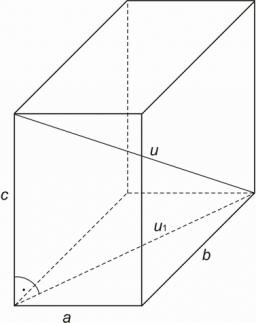# Dimensions 6264

Calculate the length of the body diagonal of a block whose dimensions are a = 5cm, b = 6cm, c = 10cm.

u =  12.6886 cm

### Step-by-step explanation:Did you find an error or inaccuracy? Feel free to write us. Thank you!

Tips to related online calculators
Tip: Our volume units converter will help you with the conversion of volume units.
Pythagorean theorem is the base for the right triangle calculator.

#### You need to know the following knowledge to solve this word math problem:

We encourage you to watch this tutorial video on this math problem: